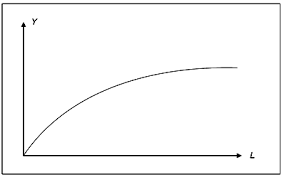# Linear functions in EconomicsLinear functions are the simplest way to define relationships between variables in economics. They are of the form:

y=a + bx

If you plot this equation on a graph, it will form a straight line. ‘a’ is the intercept on the Y-axis (that is the point on the Y-axis where the line meets the Y-axis) and ‘b’ is the slope. They are the parameters that describe the linear relationship between x and y.

Here y is the dependent variable and x is the independent variable. A linear relationship is where the change in y is always b times the change in x, irrespective of the level of x. Thus the marginal effect of x on x on y, the slope, is constant. That is why the graph is a straight line as it has a constant slope. The slope, as stated above, is the ratio of the change in y to the change in x. It thus gives us a measure of the change in y relative to the change in x. If the slope is positive, x and y have a positive relationship i.e. of x increases, y increases as well and vice versa. If the slope is negative, x and y are negatively related, i.e. they when x increases, y decreases and vice versa.

For example, let’s consider the relationship between expenditure on holidays and vacations in a linear form.

Expenditure on holidays and vacations=102 + 0.13 disposable income.

Thus when disposable income (after-tax income) increases by 1 rupee, expenditure on holidays and vacations increases by 0.13 rupees. The intercept in this equation denotes the expenditure when disposable income is zero. Here it is 102 rs. This is obviously incorrect, a family with no income will not spend on holidays. This is the drawback of a linear relationship, and hence we require non-linear equations/functions to model an economic relationship better at lower levels of income.

Here 0.13 is also the Marginal propensity to consume (spending on holidays and vacations) (MPC), that is the change in consumption caused by a change in income. As you can see, it remains constant irrespective of the level of income, which is also not true in real life. Different levels of income have different MPCs, as the share of spending on holidays and vacations in one’s change in income would be different for households with different level of income and hence the relative change in both quantities would be different as well.

•Chitranshi
April 25, 2015 6:50 am

Good work!

•Shatakshi Bhargava
May 11, 2015 3:49 pm

Mathematics has always been useful in economics and has helped in developing different models. The post is an example of this. I really liked your work 🙂

•Aikankshi Gupta
June 7, 2015 12:29 pm

nice article!

•Stephy Paul
June 17, 2015 1:34 pm

Very good article.. well done..

•Chandni Ahuja
July 29, 2015 9:18 pm

Informative.

•Vineet Agarwal
July 29, 2015 9:20 pm

Very well written

•September 2, 2015 10:22 pm

Totaly new topic 😀
A bit dificulty in understanding the concept 🙁
but it wen well after reading it for 3-4 times 😀
Suggestion make sure that you select some topic 😀 that can be easly understood my anyone 😀 without restricting your viewership 😀
GOod work 😀

•